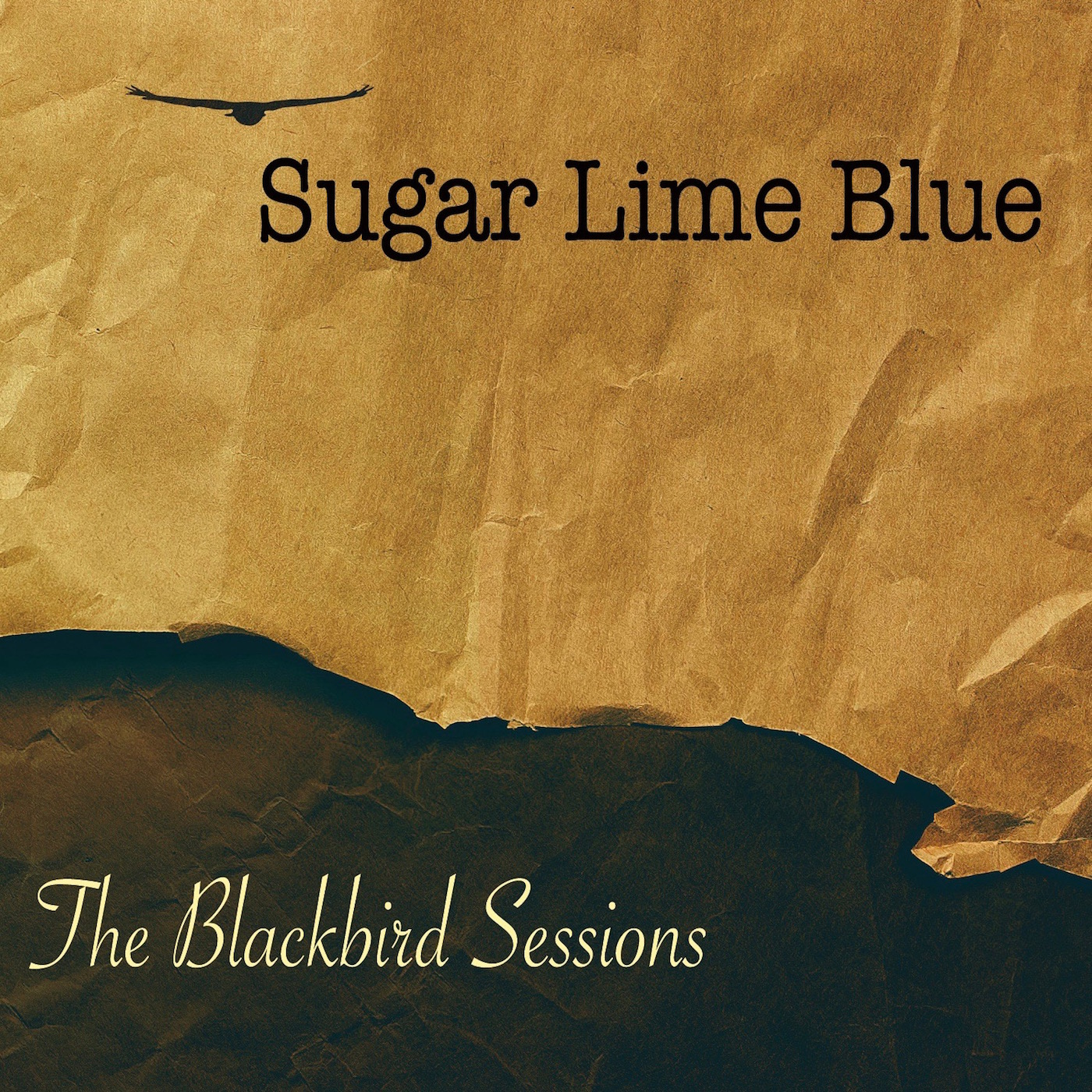Art, History, Music, Politics, and Spirituality For The Modern Alchemist – circulation in over 129 countries

# The Sign of Yuvho – Year 18,003 Begins Novemeber 5th 2015The Sign of Yuvho – Year 18,003 – Begins Novemeber 5th 2015.

There are eight months in the Calendar of Mu, sometimes referred to as the Nyarzirian Calendar. Each month consists of forty-five days, and represents a system of the eight hexagrams moving through the five elements. The Ninzuwu Shaman invokes the mudra/mantra formulae associated with each hexagram that rules a particular day after invoking the Armor of Amaterasu Ohkami. The chart below illustrates the daily Vasuh letters invoked and the ruling “deity” for the said hexagram:
1. November 5th2015 = Iut = 16th Hexagram = Aum-Hmu-Hmu
2. November 6th2015 = Aeuq = 32nd Hexagram = Tuu-Aum
3. November 7th2015 = Jimmu = 34th Hexagram = Tuu-Lewhu
4. November 8th2015 = Wosado = 40th Hexagram = Aum-Tuu-Tuu- Phe-Bnhu
5. November 9th2015 = Yuvho = 51st Hexagram = Zhee-Tuu-Aum-Shki
6. November 10th2015 = Viyah = 54th Hexagram = Zhee-Lewhu-Aum-Zhee-Zhee
7. November 11th2015 = Malukedek = 55th Hexagram = Aum-Lewhu
8. November 122015 = Raijin = 62 Hexagram = Phe-Aum
9. November 13th2015 == Entering Earth Element
10. November 14th2015 = Iut = 16thHexagram = Aum-Hmu-Hmu
11. November 15th2015 = Aeuq = 32ndHexagram = Tuu-Aum
12. November 16th2015 = Jimmu = 34thHexagram = Tuu-Lewhu
13. November 17th2015 = Wosado = 40thHexagram = Aum-Tuu-Tuu- Phe-Bnhu
14. November 18th2015 = Yuvho = 51stHexagram = Zhee-Tuu-Aum-Shki
15. November 19th2015 = Viyah = 54thHexagram = Zhee-Lewhu-Aum-Zhee-Zhee
16. November 20th2015 = Malukedek = 55thHexagram = Aum-Lewhu
17. November 21st2015 = Raijin = 62 Hexagram = Phe-Aum
18. November 22nd2015 =Entering Water Element
19. November 23rd2015 = Iut = 16thHexagram = Aum-Hmu-Hmu
20. November 24th2015 = Aeuq = 32ndHexagram = Tuu-Aum
21. November 25th2015 = Jimmu = 34thHexagram = Tuu-Lewhu
22. November 26th 2015 = Wosado = 40thHexagram = Aum-Tuu-Tuu- Phe-Bnhu
23. November 27th 2015 = Yuvho = 51stHexagram = Zhee-Tuu-Aum-Shki
24. November 28th 2015 = Viyah = 54thHexagram = Zhee-Lewhu-Aum-Zhee-Zhee
25. November 29th2015 = Malukedek = 55thHexagram = Aum-Lewhu
26. November 30th2015 = Raijin = 62 Hexagram = Phe-Aum
27. December 1st 2015 =Entering Fire Element
28. December 2nd2015 = Iut = 16thHexagram = Aum-Hmu-Hmu
29. December 3rd2015 = Aeuq = 32ndHexagram = Tuu-Aum
30. December 4th2015 = Jimmu = 34thHexagram = Tuu-Lewhu
31. December 5th2015 = Wosado = 40thHexagram = Aum-Tuu-Tuu- Phe-Bnhu
32. December 6th2015 = Yuvho = 51stHexagram = Zhee-Tuu-Aum-Shki
33. December 7th2015 = Viyah = 54thHexagram = Zhee-Lewhu-Aum-Zhee-Zhee
34. December 8th2015 = Malukedek = 55thHexagram = Aum-Lewhu
35. December 9th2015 = Raijin = 62 Hexagram = Phe-Aum
36. December 10th 2015 == Entering Air Element
37. December 11th2015 = Iut = 16thHexagram = Aum-Hmu-Hmu
38. December 12th2015 = Aeuq = 32ndHexagram = Tuu-Aum
39. December 13th2015 = Jimmu = 34thHexagram = Tuu-Lewhu
40. December 14th2015 = Wosado = 40thHexagram = Aum-Tuu-Tuu- Phe-Bnhu
41. December 15th 2015 = Yuvho = 51stHexagram = Zhee-Tuu-Aum-Shki
42. December 16th2015 = Viyah = 54thHexagram = Zhee-Lewhu-Aum-Zhee-Zhee
43. December 17th2015 = Malukedek = 55thHexagram = Aum-Lewhu
44. December 18th2015 = Raijin = 62 Hexagram = Phe-Aum
45. December 19th2015 =Entering Void Element

#### You may have missed#### What I Believe by Chris Beland#### One Breath Away by Radio Drive – An Astonishing EP